### 广汽丰田 逸致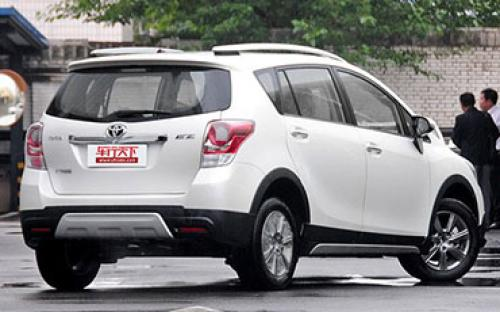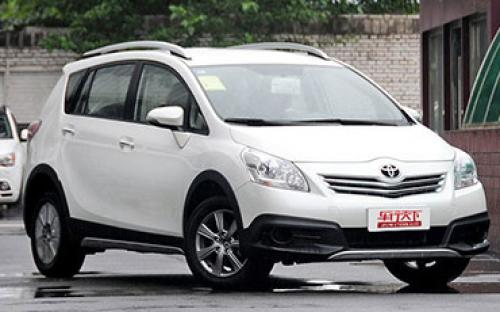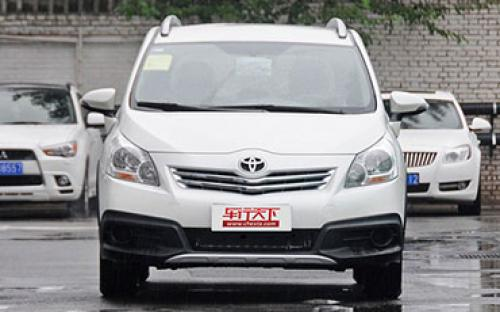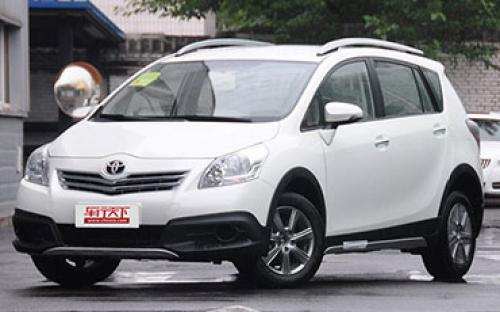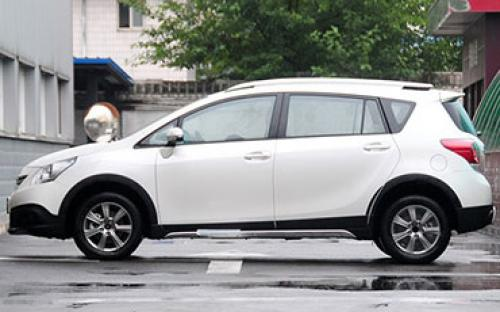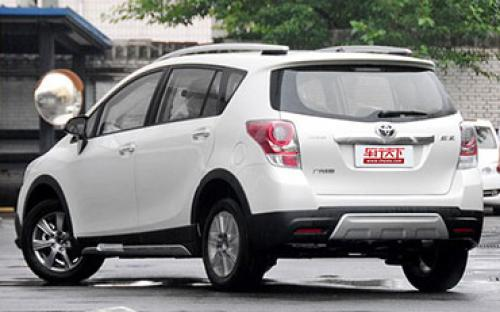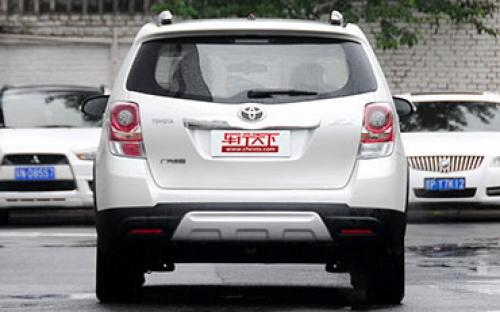6 种颜色可选2015款最低售价：16.28 万元起

4485(mm)1802(mm)1680(mm)##### 配置亮点：
• 胎压监测装置

• ISOFIX儿童座椅接口

• 车身稳定控制(ESC/ESP/DSC等)

• 电动天窗

• 定速巡航

• 后倒车雷达

• 真皮座椅

• GPS导航系统

• 氙气大灯

• 后视镜加热

• 提交

广汽丰田 逸致 绕车实拍• 广汽丰田 逸致 在售车型

排量 车型 厂商指导价 本地最低报价 购车工具
1.6L
14.98万
14.98万

15.28万
15.28万

18.98万
18.98万

1.8L
14.98万
14.98万

15.28万
15.28万

18.98万
18.98万

1.8T
星耀 180E CVT精英版 CVT无级变速
15.98万
15.98万

16.98万
16.98万

星耀 180G CVT舒适版 CVT无级变速
16.98万
16.98万

17.98万
17.98万

星耀 180G CVT豪华版 CVT无级变速
17.98万
17.98万

广汽丰田 逸致 经销商

查看更多 >>

### 广汽丰田 逸致 动力加速

逸致 0-100公里加速时间分布在 0.0-秒 属于 超跑级

动力级别 加速时间 车型

广汽丰田 逸致 视频

广汽丰田 逸致 新闻资讯

# 2014款逸致Cross实拍 重点就在外观套件

导购 超过3708次关注

逸致Cross。从名字可猜测到，它是一款以逸致为基础而推出的Cross车型。在MPV车型的基础上加入Cross元素？听着还是比较新鲜的。现在，我相信大家最为关心的是它到底...

# 2014款逸致保养解析 小保养最低376元

导购 超过4152次关注

紧凑型MPV是很符合家庭用车需求的一个分类，广汽丰田逸致就是此分类中一款比较主流的车型，价格区间为14.98-18.98万元，今天我们就来看看逸致的保养成本是怎么样的。

# 丰田逸致车展静态评测 空间组合是亮点！

试驾评测 超过4496次关注

逸致是丰田一款全新车型，它基于卡罗拉平台开发，但是并不是以轿车的身份问世，而是一款紧凑型MPV，厂商方面对其定义是：FUV（时尚多功能车），意在满足年轻人群追...

# 广汽丰田逸致跨界版上市 售16.28万元

新闻 超过2244次关注

近日，广汽丰田逸致跨界版正式上市，新车共推出一款车型，售价为16.28万元。该车基于逸致1.8L精英版5座车型的基础上升级而来。

# 逸致跨界版将于10月1日上市 增加套件

新闻 超过2317次关注

日前，我们从官方获悉，逸致跨界版车型将于10月1日正式上市，新车将引入更多跨界元素。

# 广汽丰田逸致跨界版实车图 9月份上市

新闻 超过2347次关注

近日，有媒体曝光了一组广汽丰田逸致跨界版车型的实车图。据悉，这款车将会在9月份正式上市。

猜你喜欢

﻿
• 快速找车
• 选择品牌
• 选择品牌
• A  奥迪
• A  阿斯顿·马丁
• A  阿尔法·罗密欧
• B  宝沃
• B  布加迪
• B  巴博斯
• B  保时捷
• B  宾利
• B  奔驰
• B  宝马
• B  本田
• B  别克
• B  标致
• B  比亚迪
• B  宝骏
• B  北汽制造
• B  北汽新能源
• B  北汽幻速
• B  北汽威旺
• B  北京汽车
• B  奔腾
• B  北汽绅宝
• C  长安
• C  长安商用
• C  长城
• C  昌河
• D  大众
• D  道奇
• D  DS
• D  东南
• D  东风风神
• D  东风风行
• D  东风小康
• D  东风风度
• D  东风
• F  福特
• F  丰田
• F  菲亚特
• F  法拉利
• F  福田
• F  福迪
• F  福汽启腾
• G  观致
• G  广汽传祺
• G  广汽吉奥
• G  GMC
• H  红旗
• H  汉腾汽车
• H  哈弗
• H  哈飞
• H  海格
• H  海马
• H  华颂
• H  黄海
• H  华泰
• H  恒天
• J  吉利汽车
• J  捷豹
• J  Jeep
• J  江淮
• J  江铃
• J  金杯
• J  九龙
• J  金旅
• K  凯翼
• K  凯迪拉克
• K  克莱斯勒
• K  科尼塞克
• K  卡威
• K  开瑞
• L  路虎
• L  林肯
• L  劳斯莱斯
• L  兰博基尼
• L  雷克萨斯
• L  铃木
• L  雷诺
• L  理念
• L  力帆
• L  莲花汽车
• L  猎豹
• L  路特斯
• L  陆风
• M  马自达
• M  MG
• M  MINI
• M  玛莎拉蒂
• M  摩根
• M  迈凯轮
• N  纳智捷
• O  欧宝
• O  讴歌
• O  欧朗
• Q  奇瑞
• Q  起亚
• Q  启辰
• R  日产
• R  荣威
• R  瑞麒
• S  三菱
• S  斯威汽车
• S  萨博
• S  smart
• S  斯柯达
• S  斯巴鲁
• S  思铭
• S  双龙
• S  上汽大通
• S  双环
• T  特斯拉
• T  腾势
• W  沃尔沃
• W  五菱汽车
• W  五十铃
• W  威兹曼
• W  威麟
• X  现代
• X  雪佛兰
• X  雪铁龙
• X  西雅特
• Y  一汽
• Y  英菲尼迪
• Y  英致
• Y  依维柯
• Y  野马汽车
• Y  永源
• Z  众泰
• Z  中华
• Z  中兴
• Z  知豆
• 选择车系
• 选择车系
• 车型对比
• 选择品牌
• 选择品牌
• A  奥迪
• A  阿斯顿·马丁
• A  阿尔法·罗密欧
• B  宝沃
• B  布加迪
• B  巴博斯
• B  保时捷
• B  宾利
• B  奔驰
• B  宝马
• B  本田
• B  别克
• B  标致
• B  比亚迪
• B  宝骏
• B  北汽制造
• B  北汽新能源
• B  北汽幻速
• B  北汽威旺
• B  北京汽车
• B  奔腾
• B  北汽绅宝
• C  长安
• C  长安商用
• C  长城
• C  昌河
• D  大众
• D  道奇
• D  DS
• D  东南
• D  东风风神
• D  东风风行
• D  东风小康
• D  东风风度
• D  东风
• F  福特
• F  丰田
• F  菲亚特
• F  法拉利
• F  福田
• F  福迪
• F  福汽启腾
• G  观致
• G  广汽传祺
• G  广汽吉奥
• G  GMC
• H  红旗
• H  汉腾汽车
• H  哈弗
• H  哈飞
• H  海格
• H  海马
• H  华颂
• H  黄海
• H  华泰
• H  恒天
• J  吉利汽车
• J  捷豹
• J  Jeep
• J  江淮
• J  江铃
• J  金杯
• J  九龙
• J  金旅
• K  凯翼
• K  凯迪拉克
• K  克莱斯勒
• K  科尼塞克
• K  卡威
• K  开瑞
• L  路虎
• L  林肯
• L  劳斯莱斯
• L  兰博基尼
• L  雷克萨斯
• L  铃木
• L  雷诺
• L  理念
• L  力帆
• L  莲花汽车
• L  猎豹
• L  路特斯
• L  陆风
• M  马自达
• M  MG
• M  MINI
• M  玛莎拉蒂
• M  摩根
• M  迈凯轮
• N  纳智捷
• O  欧宝
• O  讴歌
• O  欧朗
• Q  奇瑞
• Q  起亚
• Q  启辰
• R  日产
• R  荣威
• R  瑞麒
• S  三菱
• S  斯威汽车
• S  萨博
• S  smart
• S  斯柯达
• S  斯巴鲁
• S  思铭
• S  双龙
• S  上汽大通
• S  双环
• T  特斯拉
• T  腾势
• W  沃尔沃
• W  五菱汽车
• W  五十铃
• W  威兹曼
• W  威麟
• X  现代
• X  雪佛兰
• X  雪铁龙
• X  西雅特
• Y  一汽
• Y  英菲尼迪
• Y  英致
• Y  依维柯
• Y  野马汽车
• Y  永源
• Z  众泰
• Z  中华
• Z  中兴
• Z  知豆
• 选择车系
• 选择车系
• 选择车型
• 选择车型
• 意见反馈